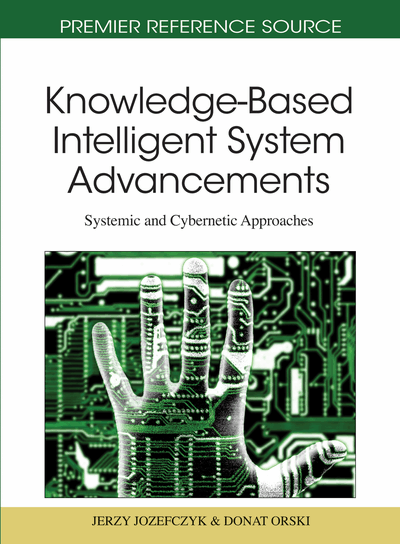# Probabilistic Temporal Network for Numeric and Symbolic Time Information

Malek Mouhoub (University of Regina, Canada) and Jia Liu (University of Regina, Canada)
DOI: 10.4018/978-1-61692-811-7.ch004
Available
\$29.50
No Current Special Offers

## Abstract

We propose a probabilistic extension of Allen’s Interval Algebra for managing uncertain temporal relations. Although previous work on various uncertain forms of quantitative and qualitative temporal networks have been proposed in the literature, little has been addressed to the most obvious type of uncertainty, namely the probabilistic one. More precisely, our model adapts the probabilistic Constraint Satisfaction Problem (CSP) framework in order to handle uncertain symbolic and numeric temporal constraints. In a probabilistic CSP, each constraint C is given a probability of its existence in the real world. There is thus more than one CSP to solve as opposed to the traditional CSP where no such uncertainties exist. In a probabilistic temporal CSP, since we use the Interval Algebra where a constraint is a disjunction of Allen primitives, the probability is assigned to each of these Allen primitives rather than to the temporal constraint. This means that a probabilistic temporal CSP involves many possible temporal CSPs, each with a probability of its existence. Solving a probabilistic temporal CSP consists of finding a scenario that has the highest probability to be the solution for the real world. This is an optimization problem that we solve using a branch and bound algorithm we propose and involving constraint propagation. Experimental study conducted on randomly generated temporal problems demonstrates the efficiency in time of our solving method. In the case of uncertain numeric constraints, our TemPro framework for handling numeric and symbolic temporal constraints is extended to handle uncertain domains. An algorithm for dividing domains into non-overlapping areas is proposed. This algorithm guarantees that the generated possible worlds do not intersect. Probable worlds are then constructed by combining these areas. A new branch and bound algorithm, we propose, is finally applied to find the most robust solution.
Chapter Preview
Top

## Introduction

A Constraint Satisfaction Problem (CSP) (Dechter, 2003, Haralick, 1980, Mackworth, 1977) is a general model for many problems in the real world. A CSP is defined as a tuple < X, D, C > where X is a set of variables, D their domains of values and C the set of constraints restricting the values that the variables can simultaneously take. A Temporal Constraint Satisfaction Problem (Allen, 1983, Dean 1989, Morris 2000, van Beek, 1992, Vilan 1986) is a special type of CSPs that handles temporal information. There are many applications of Temporal CSPs, such as scheduling (Baptiste, 1995), planning (Golumbic 1993, Tsamardinos 2003,Vidal 1996), natural language processing (Hwang, 1994), temporal database (Dean, 1989) and molecular biology (Golumbic 1993). These applications usually come with uncertain factors from the real world. For example, when we are trying to schedule a plan for traveling, the aircraft we take may not arrive at the destination on time. When we are allocating tasks among processors, some processors may break down. With these uncertain factors, some solutions may be better suited for the environment than others. However, all the solutions are treated equally in the traditional temporal CSPs without uncertainty. Our goal is to find the most robust solution that is associated with the highest probability to satisfy all the constraints in the uncertain temporal CSP.

Temporal information can be symbolic or numeric. In (Mouhoub, 2004), we have proposed the TemPro framework for managing discrete numeric and symbolic temporal information. The variables in TemPro are events associated with temporal intervals. The domain of each event is the discrete and finite set of the possible numeric intervals the event can take. Constraints in TemPro specify the possible temporal relationships among events. Solving a problem represented in TemPro (that we call Temporal Constraint Satisfaction Problem (TCSP)) is to find an assignment of one numeric interval to each event, in such a way that every constraint is satisfied. In symbolic temporal CSPs, the variables are the relations between time points or time intervals. In the case of relations between time intervals, the constraint network is called Interval Algebra (IA) network (Allen, 1983). In the case of an IA network these relations are disjunctions of Allen primitives. Allen primitives are the thirteen possible relations between a pair of numeric intervals. Solving an IA network consists of finding an assignment of a possible Allen primitive to each disjunctive relation such that the entire IA network is consistent.

## Complete Chapter List

Search this Book:
Reset